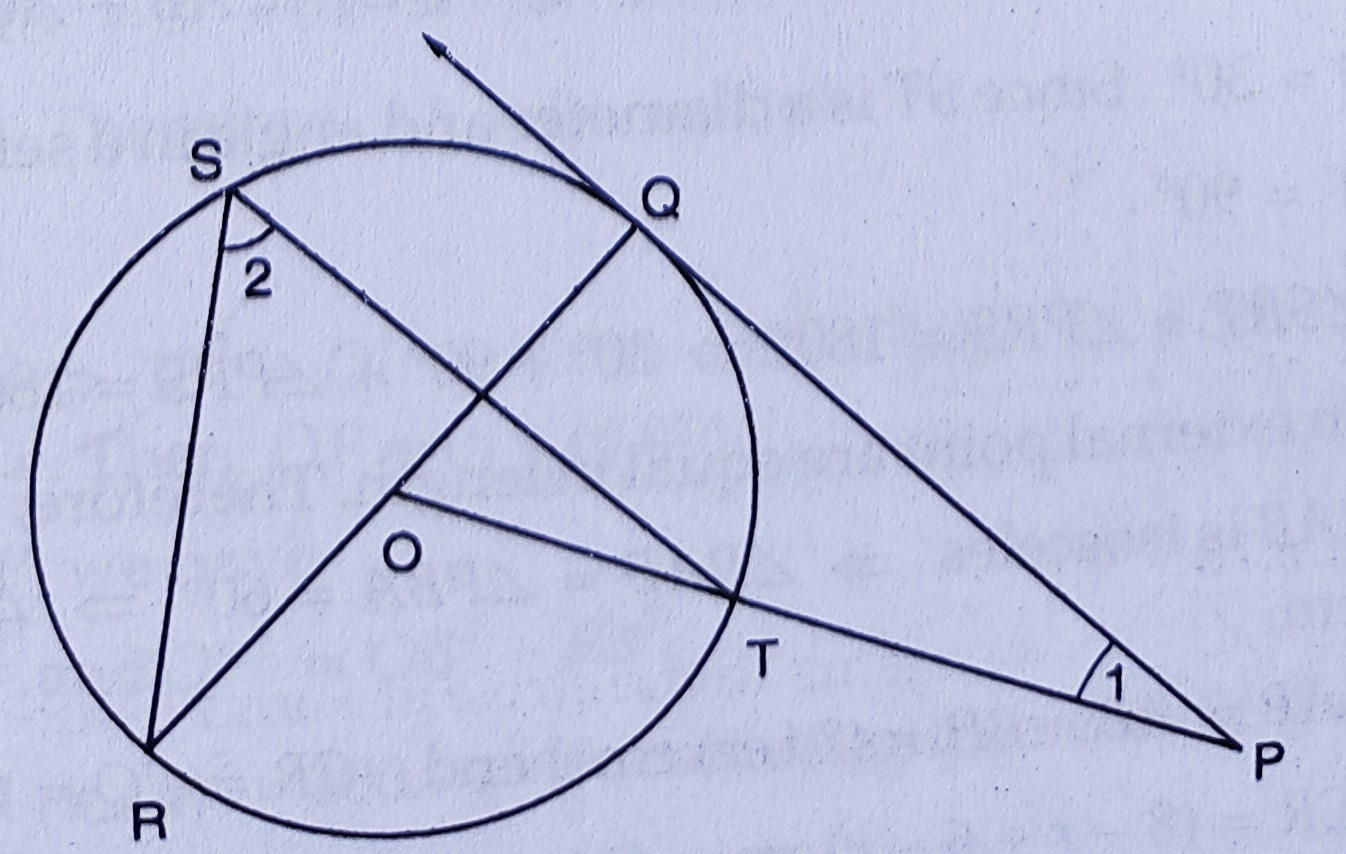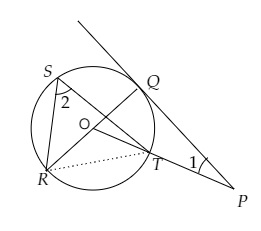"
">

# In the figure, $P Q$ is a tangent from an external point $P$ to a circle with centre $O$ and $O P$ cuts the circle at $T$ and $Q O R$ is a diameter. If $\angle P O R=130^{\circ}$ and $S$ is a point on the circle, find $\angle 1+\angle 2$."

Given:

In the figure, $P Q$ is a tangent from an external point $P$ to a circle with centre $O$ and $O P$ cuts the circle at $T$ and $Q O R$ is a diameter.

$\angle P O R=130^{\circ}$ and $S$ is a point on the circle.

To do: We have to find $\angle 1+\angle 2$.

Solution:Join $RT$.

$\angle POR = 130^o$

$\angle POQ = 180^o- \angle POR$ $= 180^o - 130^o$

$= 50^o$

$PQ$ is a tangent to the circle.

$\angle PQO = 90^o$

In $\triangle POQ$,

$\angle POQ + \angle PQO + \angle QPO = 180^o$

$50^o + 90^o + \angle 1 = 180^o$

$\angle 1 = 180^o - 140^o$

$\angle 1 = 40^o$

In $\triangle RST$,

$\angle RST = \frac{1}{2} \angle ROT$      (Angle subtended by an arc at the centre of a circle is twice the angle subtended at any point on the circumference)

$\angle 2 = \frac{1}{2} \times 130^o$

$= 65^o$

Therefore,

$\angle 1 + \angle 2 = 40^o + 65^o$

$= 105^o$

Therefore, $\angle 1+\angle 2 = 105^o$.

Updated on: 10-Oct-2022

40 Views